Function Repository Resource:

# PermutationCountByCycleLength

Calculate the number of permutations of the specified cycle length counts

Contributed by: Wolfram Staff (original content by Sriram V. Pemmaraju and Steven S. Skiena)
 ResourceFunction["PermutationCountByCycleLength"][{λ1,λ2,…}] gives the number of permutations of the specified cycle length counts {λ1,λ2,…}.

## Details and Options

The number of permutations with a given list of cycle length counts {λ1,λ2,} is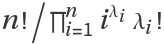, where n is the length of the list.
As the list {λ1,λ2,} is also known as the permutation type, ResourceFunction["PermutationCountByCycleLength"] returns the number of permutations by type.

## Examples

### Basic Examples (1)

The number of 6-permutations that have two 1-cycles and three 2-cycles:

 In:=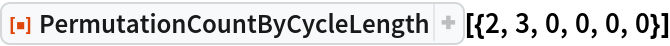Out=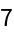### Properties and Relations (4)

There are (n-1)! n-permutations with one cycle:

 In:=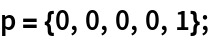In:=Out=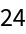In:=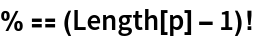Out=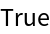There are n(n-2)! n-permutations with one singleton cycle and one (n-1)-cycle:

 In:=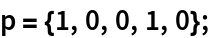In:=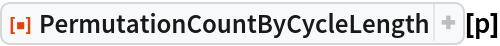Out=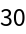In:=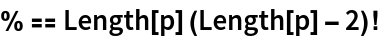Out=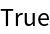The CycleLengthCountList resource function is a list of all possible cycle length counts (permutation types) into which an n-permutation can be partitioned:

 In:=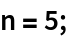In:=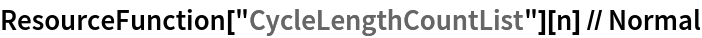Out=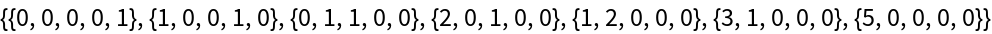Count permutations by type:

 In:=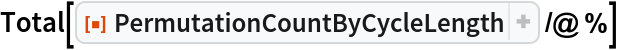Out=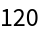As expected, there are n! permutations:

 In:=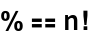Out=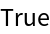Use PermutationCountByCycleLength to tally the number of permutations of all the possible types:

 In:=Out=Tallying CycleLengthCounts in the Permutations list gives the same result:

 In:=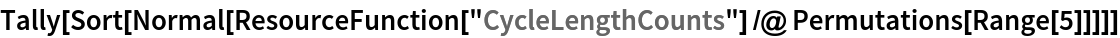Out=In:=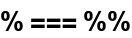Out=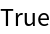## Version History

• 1.0.0 – 09 July 2020